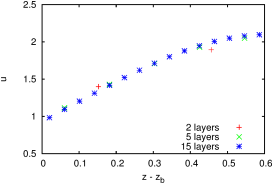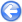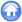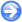### 12.2PASS: Transcritical flow over a bump

Author
Stéphane Popinet
Command
sh shock.sh
Version
120717
Required files
shock.sh error.ref
Running time
33 seconds

This is a classical test case for shallow-water solvers. Water flowing over a bump goes from subcritical through supercritical over the bump and back to subcritical. Figure 146 illustrates the stationary solution with a shock. Figure 147 gives the convergence with resolution. The analytical solution was obtained thanks to the SWASHES library .

 Figure 146: Numerical and analytical solutions for transcritical flow over a bump.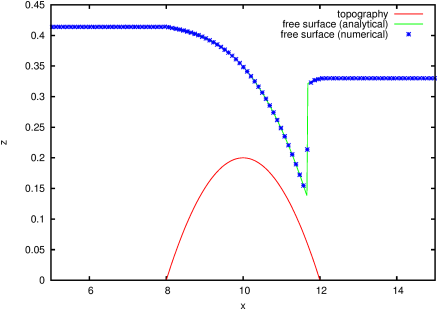Figure 147: Convergence of the error between the numerical and analytical solution with the spatial resolution.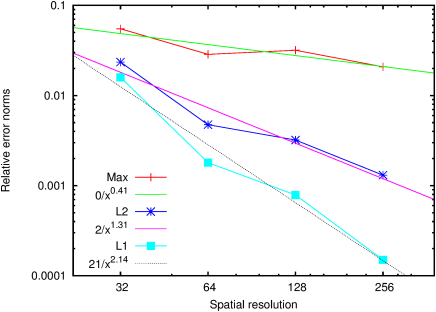#### 12.2.1PASS: Transcritical flow with multiple layers

Author
Stéphane Popinet
Command
sh layered.sh
Version
120717
Required files
layered.sh uprof.awk field.awk prof.ref
Running time
1 minutes 52 seconds

This is meant to replicate the multilayer test case of [4, 5]. The vertical viscosity is set to ν=0.01 m2/s and the Navier condition at the bottom is given by the Strickler relation

k(h,U)=
 g S2h1/3
||U||

with S=25 m1/3/s, the Strickler coefficient, h the water depth and U the depth-averaged velocity.

The stationary solutions are summarised in the figures below. They can be compared to Figure 9 of  and Figure 8 of . They agree more or less although our Strickler coefficient is lower (i.e. we need a larger explicit friction to match [4, 5]).

 Figure 148: Free surface and topograghy.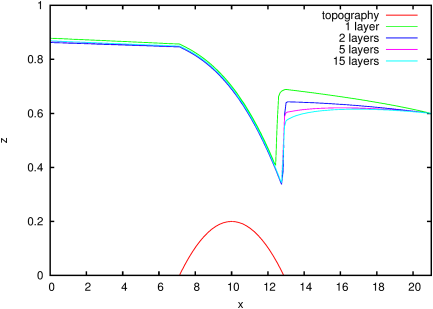Figure 149: Horizontal velocity field (15 layers).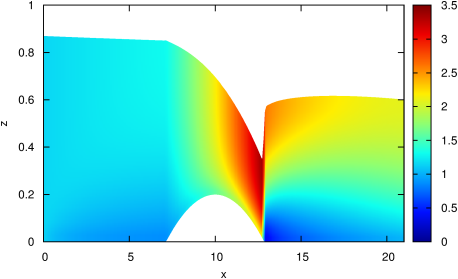Figure 150: Velocity profiles at (a) x=10, (b) x=15, (c) x=20.
 (a)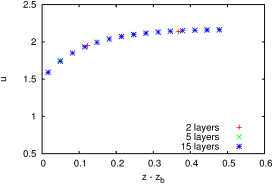(b)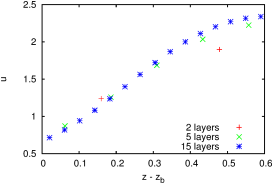(c)